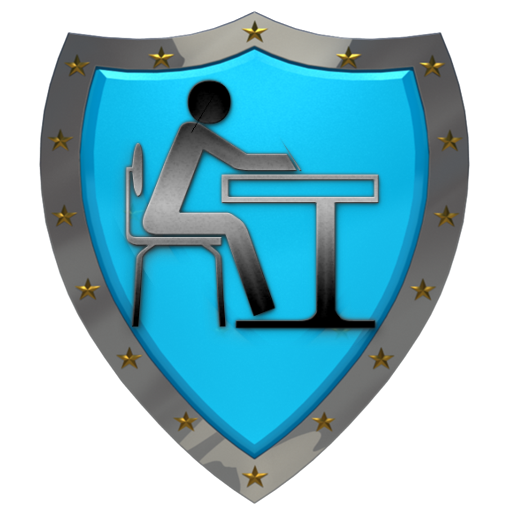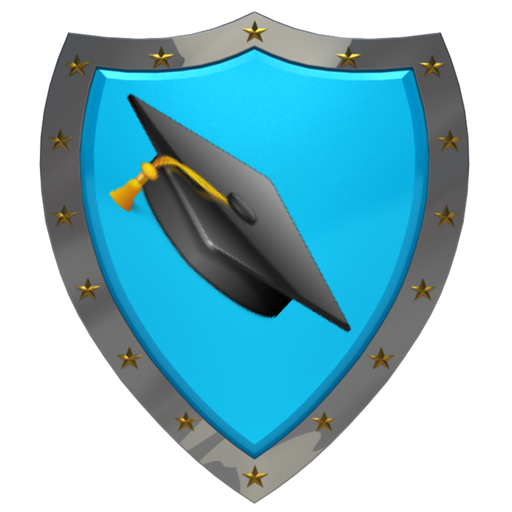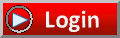Avatar Virtual Learning"Lowering College Education Costs for Everyone"CoursesTesting OutMy StuffFAQsAboutCalculus (SMA-002)
The Calculus examination covers skills and concepts that are usually taught in a one-semester college course in calculus. The content of each examination is approximately 60% limits and differential calculus and 40% integral calculus. Algebraic, trigonometric, exponential, logarithmic and general functions are included. The exam is primarily concerned with an intuitive understanding of calculus and experience with its methods and applications. Knowledge of preparatory mathematics is assumed, including algebra, geometry, trigonometry and analytic geometry.
Current status of course:
 In Development

Chemistry (SMA-003)
The Chemistry examination covers material that is usually taught in a one-year college course in general chemistry. Understanding of the structure and states of matter, reaction types, equations and stoichiometry, equilibrium, kinetics, thermodynamics, and descriptive and experimental chemistry is required, as is the ability to interpret and apply this material to new and unfamiliar problems. During this examination, an online scientific calculator function and a periodic table are available as part of the testing software.
Current status of course:
 In Development

College Mathematics (SMA-005)
The College Mathematics examination covers material generally taught in a college course for non-mathematics majors and majors in fields not requiring knowledge of advanced mathematics. Questions on the College Mathematics examination require you to demonstrate the ability to solve routine, straightforward problems and non-routine problems that require an understanding of concepts and the application of skills and concepts. In addition, you need to demonstrate understanding of topics like: sets, logic, real number systems, functions and graphs, probability and statistics, as well as topics in algebra and geometry.
Current status of course:
 In Development

Precalculus (SMA-007)
The Precalculus examination assesses the mastery of skills and concepts required for success in a first-semester calculus course. A large portion of the exam tests your understanding of functions and their specific properties: linear, quadratic, absolute value, square root, polynomial, rational, exponential, logarithmic, trigonometric, inverse trigonometric and piecewise-defined functions. Questions on the exam will present these types of functions symbolically, graphically, verbally or in tabular form. A solid understanding of these types of functions is at the core of all precalculus courses, and it is a prerequisite for enrolling in calculus and other college-level mathematics courses.
Current status of course:
 In Development